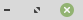https://dev.webpages.dk/PHP Use of IF and ELSE
PHP How to Use IF and ELSE:The IF and ELSE statements. They are used to see if a variable is of a certain value, and then react on it. And if the variable is not that value then it will execute what is in the ELSE statement.

See the code in the 'PHP' tab to the right.

### IF and ELSE

How do you Use IF and ELSE in PHP?

In this posting we will look shortly at IF and ELSE in PHP.
In the code below you can see two textfields and a simple checkbox and a submitbutton.

The content of the textfields are send to the screen when submitting, and if you have ticked the checkbox you will put a string into the variable \$check. Then the whole page is send to the browser, and depending on if the box was ticked or not the message is shown along the firstname and lastname of your contact. If the box was checked we write a message into the variable, if not we just write an empty string.

We read the checkbox value using \$_POST['member'], and with IF we simply assign a string to the variable if the checkbox was ticked. In the checkbox HTML form field you can see that the value is set to 'on', this is what we look for. If you have other input types in your form, then what you look for are these VALUES.
We also use ELSE. That is simply if the \$_POST returns any thing else than 'on'.

### HTML

``````<HTML><BODY>
<FORM METHOD='POST' ACTION=''>
<TABLE>

<TR>
<TD></TD>
<TD><?php echo "Your contact is: " . \$Firstname . " " . \$Lastname . "<BR/>" . \$check;?><BR /></TD>
</TR>

<TR>
<TD>Firstname: </TD>
<TD><INPUT TYPE='text' NAME='firstname' VALUE='" . \$Firstname . "'>
</TD>
</TR>

<TR>
<TD>Lastname: </TD>
<TD><INPUT TYPE='text' NAME='lastname' VALUE='" . \$Lastname . "'>
</TD>
</TR>

<TR>
<TD>Member: </TD>
<TD><INPUT TYPE='checkbox' NAME='member' VALUE='on'></TD>
</TR>

<TR>
<TD></TD>

</TR>
</TABLE>
</FORM>
</BODY></HTML>``````

### PHP

``````<?php
\$Firstname = \$_POST['firstname'];
\$Lastname = \$_POST['lastname'];
\$Member = \$_POST['member'];

if ( \$Member == 'on' ) { \$check = 'She is a member'; }
else { \$check = ''; }
?>``````
 Icons made by Freepik from www.flaticon.com Here is an example of how to use IF and ELSE in PHP. 15:38:28